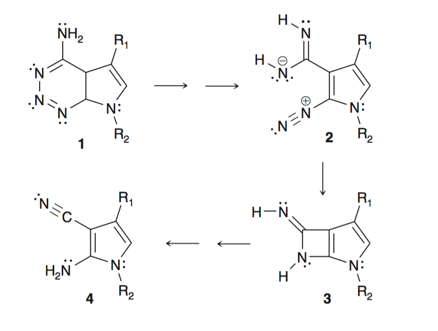# Problem: Compound 1 undergoes a thermal elimination of nitrogen at C to form nitrile  4 (Org. Lett. 1999, 1, 537–539). One proposed subsequently refuted) mechanism for this transformation involves intermediates 2 and 3:  (a) Draw curved arrows that show the conversion of  2 to 3.

###### Problem Details

Compound 1 undergoes a thermal elimination of nitrogen at C to form nitrile  4 (Org. Lett. 1999, 1, 537–539). One proposed subsequently refuted) mechanism for this transformation involves intermediates 2 and 3

(a) Draw curved arrows that show the conversion of  2 to 3.What scientific concept do you need to know in order to solve this problem?

Our tutors have indicated that to solve this problem you will need to apply the Reaction Mechanism concept. You can view video lessons to learn Reaction Mechanism. Or if you need more Reaction Mechanism practice, you can also practice Reaction Mechanism practice problems.

What is the difficulty of this problem?

Our tutors rated the difficulty ofCompound 1 undergoes a thermal elimination of nitrogen at C ...as high difficulty.

How long does this problem take to solve?

Our expert Organic tutor, Chris took 1 minute and 36 seconds to solve this problem. You can follow their steps in the video explanation above.

What textbook is this problem found in?

Our data indicates that this problem or a close variation was asked in Organic Chemistry - Klein 2nd Edition. You can also practice Organic Chemistry - Klein 2nd Edition practice problems.